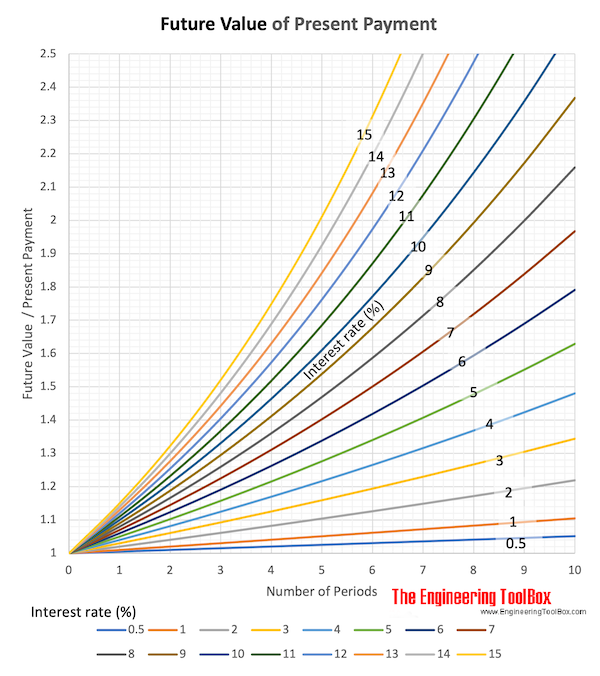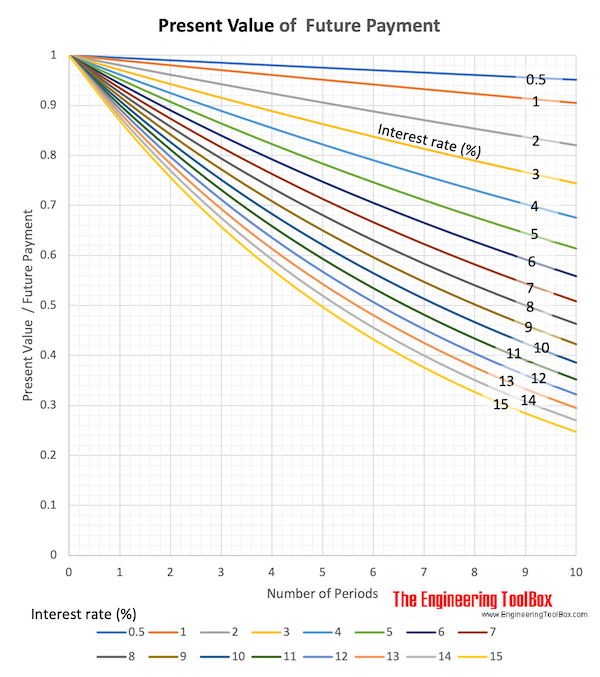Engineering ToolBox - Resources, Tools and Basic Information for Engineering and Design of Technical Applications!

# Interest Formulas

## Future value of single cash flow.

### Single Cash Accumulation - Future Value

The accumulated value of a present sum invested at a given interest rate after some time can be expressed as

F = P (1 + i)n         (1)

where

F = accumulated value in the future- Future Value

P = principal or present sum invested

i = interest rate per period

n = number of interest periods

The factor "(1 + i)n" is known as the "single payment compound amount factor".

#### Example - Accumulated amount

An amount of 1000 is invested at interest rate 10% (0.1) per year for 10 years. The accumulated amount can be calculated like

F = 1000 (1 + 0.1)10

= 2594### Discounting Process - Present Value

The Present Value - or Present Worth - of a future cash flow can be calculated with the discounting process and is expressed like

P = F / (1 + i)n         (2)

where

P = present value

F = future cash flow

i = discount rate or discounting factor

n = numbers of periods

The factor "1 / (1 + i)n" is known as the "single payment present worth factor".

#### Example - The present value of a future sum

A sum of 1000 is paid after 10 years. The discount rate is 10% (0.1) per year. The present value of the future payment can be calculated like

P = 1000 / (1 + 0.1)10

= 386## Related Topics

• ### Economics

Engineering economics - cash flow diagrams, present value, discount rates, internal rates of return - IRR, income taxes, inflation.

## Related Documents

• ### Cash Flow Diagrams

The future value of money.
• ### Discrete Compounding Cash Flow Formulas

Discrete payments compounding equations and online calculators .
• ### Interest Rate

Interest rate is the cost of money.
• ### Internal Rate of Return - IRR

Internal Rate of Return (IRR) - the break-even interest rate.
• ### Net Present Worth (NPW) of a Cash Stream

The value of a stream of payments is called the Net Present Worth (NPW).
• ### Present Value

The value of money in the future is the Present Value.

## Engineering ToolBox - SketchUp Extension - Online 3D modeling!

Add standard and customized parametric components - like flange beams, lumbers, piping, stairs and more - to your Sketchup model with the Engineering ToolBox - SketchUp Extension - enabled for use with older versions of the amazing SketchUp Make and the newer "up to date" SketchUp Pro . Add the Engineering ToolBox extension to your SketchUp Make/Pro from the Extension Warehouse !

We don't collect information from our users. More about

## Citation

• The Engineering ToolBox (2008). Interest Formulas. [online] Available at: https://www.engineeringtoolbox.com/interest-formulas-d_1230.html [Accessed Day Month Year].

Modify the access date according your visit.

9.27.11